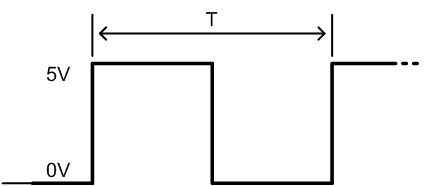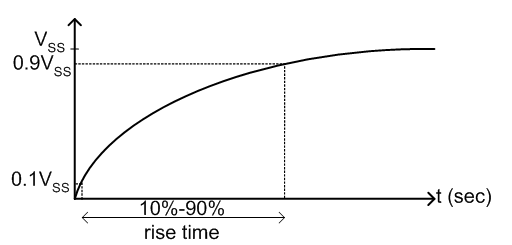### Prelab 3: Square Waves and RC Time Constants

1. For the RC circuit of figure 1 of the lab:

1. (a) What is the theoretical time constant t ("tau") for the circuit?

(b) Sketch one period of the output waveform on top of the input square wave with period T shown, where:

i)  T = 10tii)  T = tiii) T = 0.1t(c) Calculate the relationship between the 10% to 90% rise time (as shown below) and the time constant t for the circuit's step response.Hint: you'll need to solve for the step response of the circuit.